Students can Download Maths Chapter 4 Statistics Ex 4.2 Questions and Answers, Notes Pdf, Samacheer Kalvi 8th Maths Book Solutions Guide Pdf helps you to revise the complete Tamilnadu State Board New Syllabus and score more marks in your examinations.

## Tamilnadu Samacheer Kalvi 8th Maths Solutions Term 3 Chapter 4 Statistics Ex 4.2

Question 1.
Which of the following data can be represented in a histogram?

1. The number of mountain climbers in the age group 20 to 60.
2. Production of cycles in different years.
3. The number of students in each class of a school.
4. The number votes polled from 7 am to 6 pm in an election.
5. The wickets fallen from 1 over to 50th over in a one day cricket match.

1. Yes
2. No
3. No
4. Yes
5. Yes

Question 2.
Fill in the blanks

1. The area of the rectangles are proportional to the _______ given.
2. The total area of the histogram is _______ to the total frequency of the given data.
3. _______ is a graphical representation of continuous frequency distribution with rectangles.
4. Histogram is a graphical representation of _______ data.

1. frequency
2. proportional
3. Histogram
4. groupedQuestion 3.
In a village, there are 570 people who have cell phones. An NGO survey their cell phone usage. Based on this survey a histogram is drawn. Answer the following questions.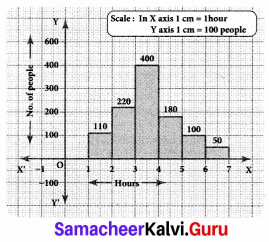(i) How many people use the cell phone for less than 3 hours?
Solution:
330 people (110+ 220)

(ii) How many of them use the cell phone for more than 5 hours?
Solution:
150 of them (100 + 50)

(iii) Are people using cell phone for less than 1 hour?
Solution:
No.

(iv) Give your suggestions on the data.
Solution:
People should minimise the usage of cell phones.Question 4.
Draw a histogram for the following data.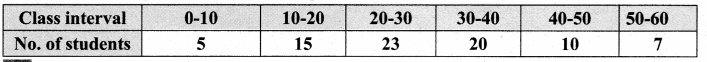Solution:
The given data is continuous frequency distribution taking class intervals on X axis and No. of students on Y-axis, the histogram is given below.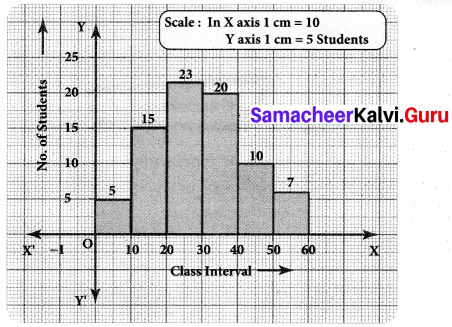Question 5.
Construct a histogram from the following distribution of total marks of 40 students in a class.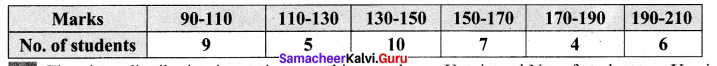Solution:
The given distribution is continuous taking marks on X axis and No. of students on Y-axis the histogram is constructed.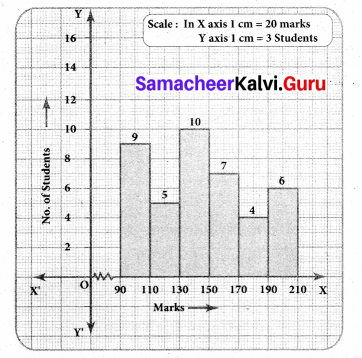Question 6.
The distribution of heights (in cm) of 100 people is given below. Construct a histogram and the frequency polygon imposed on it.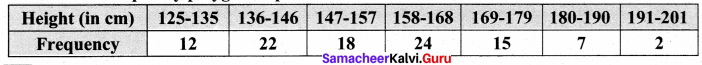Solution:
The given distribution is discontinuous.
Converting into continuous distribution we have
Lower boundary = lower limit – $$\frac { 1 }{ 2 }$$ (gap between the adjacent class interval)
= 125 – $$\frac { 1 }{ 2 }$$ (1) = 124.5
Upper boundary = Upper limit + $$\frac { 1 }{ 2 }$$ (gap between adjacent class interval)
= 135 + $$\frac { 1 }{ 2 }$$ = 135.5
∴ The new frequency table is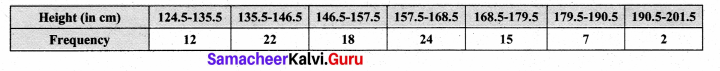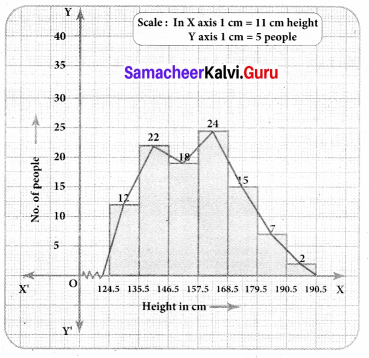+Question 7.
In a study of dental problem, the following data were obtained.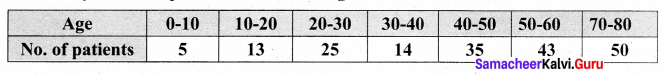Represent the above data by a frequency polygon.
Solution:
Finding the midpoints of the class interval we get.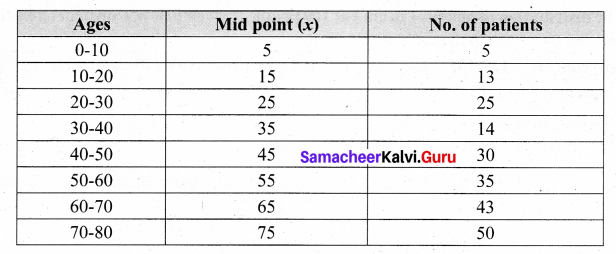The points to be plotted are A (5,5), B (15, 13), C (25, 25), D (35, 14), E (45, 30), G (65, 43.), H (75, 50) to obtain the frequency polygon ZABCDEFGHI.
Where I imagined class between 80 and 90.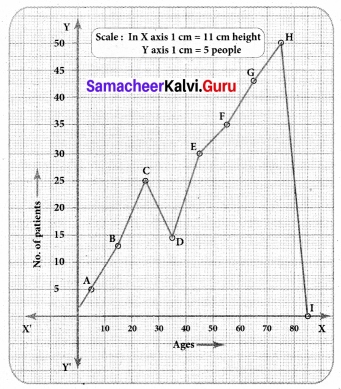Question 8.
The marks obtained by 50 students in Mathematics are given below (i) Make a frequency distribution table taking a class size of 10 marks (ii) Draw a histogram and a frequency polygon.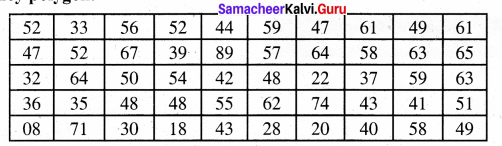Solution:
Maximum marks obtained = 89
Minimum marks obtained = 08
Range = Maximum marks – Minimum marks = 89 – 08 = 81
Taking the class size = 10, then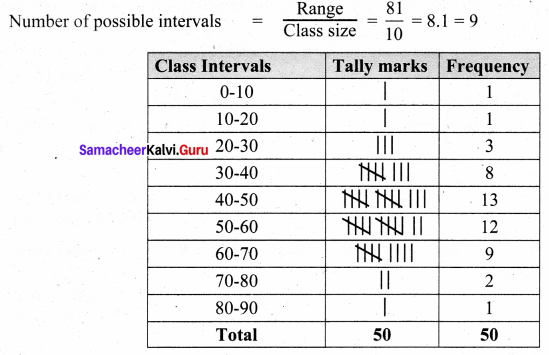Now we have the continuous frequency table.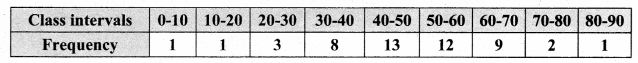We will draw the histogram taking class interval in x axis and frequency in y axis as follows.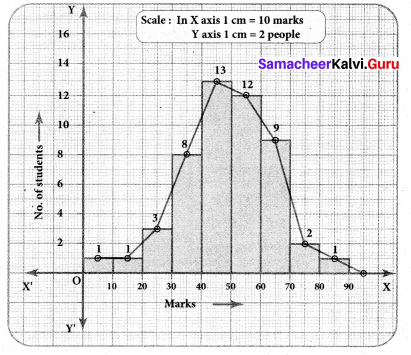Objective Type Questions

Question 1.
Data is a collection of _______
(a) numbers
(b) words
(c) measurements
(d) all the three
(d) all the three

Question 2.
The number of times an observation occurs in the given data is called _______
(a) tally marks
(b) data
(c) frequency
(d) none of these
(c) frequency

Question 3.
The difference between the largest value and the smallest value of the given data is _______
(a) range
(b) frequency
(c) variable
(d) none of these
(a) rangeQuestion 4.
The data that can take values between a certain range is called _______
(a) ungrouped
(b) grouped
(c) frequency
(d) none of these
(b) grouped

Question 5.
Inclusive series is a _______ series
(a) continuous
(b) discontinuous
(c) both
(d) none of these
(b) discontinuous

Question 6.
In a class interval the upper limit of one class is the lower limit of the other class. This is _______ series.
(a) Inclusive
(b) exclusive
(c) ungrouped
(d) none of these
(b) exclusive

Question 7.
The graphical representation of un grouped data is _______
(a) histogram
(b) frequency polygon
(c) pie chart
(d) all the threeQuestion 8.
Histogram is a graph of a _______ frequency distribution.
(a) continuous
(b) discontinuous
(c) discrete
(d) none of these
(a) continuous

Question 9.
A _______ is a line graph for the graphical representation of the continuous frequency distribution.
(a) frequency polygon
(b) histogram
(c) pie chart
(d) bar graph